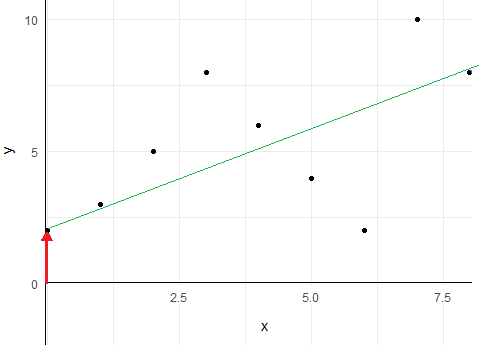# Intercept in multivariate analysis

Modified on Mon, 23 Nov 2020 at 11:06 AM

# What is the intercept ?

The intercept is the point where the regression line crosses the y axis i.e. when the explanatory variables are all equal to zero.The red arrow indicates the point which is called intercept

# Why do I have this parameter in my model ?

In a regression model, there are always two parts which are computed to predict the variable one wants to explain: the explanatory part using the explanatory variables chosen and the residual part representing the part of the response which cannot be explained by the explanatory variables used.

This second part is usually called "residuals term". This term could be positive or negative. If this term is positive, the model trends to overestimate the response; if this term is negative, the model trends to underestimate the response. The role of the intercept is then to counterbalance the residuals term to unsure the model to be equal to zero when the explanatory variables are all equal to zero (even if this event could never arrive in reality), meaning that the results of the model will not be due to the residuals term but only to the explanatory variables implied.

It is possible to perform a model without intercept. In fact, it is uncommon and should be only make when one is sure that when the values of all explanatory variables are equal to zero, the intercept in reality should be zero. That implies that the true relationship between the variable to explain and the explanatory variables is already at least partially known. In practice, it rarely happens in medicine.

# How can I interpret the value of the coefficient of the intercept ?

Usually, interpret the value of the coefficient of the intercept makes no sens because it is only interesting to perfect the model. The only situation of interest could be when the coefficients of all of the explanatory variables are near to zero and the coefficient of the intercept is high: in this case, one could think that the model computed with the explanatory  variables chosen doesn't explain as much the variable to explain. Thus, either the relationship between the response variable and the explanatory variables isn't linear, or others explanatory variables should better explain the response variable.

# How can I interpret the p-value of the coefficient of the intercept ?

The p-value of the intercept indicates what would be the percentage of samples that will have a coefficient as far away from 0 or more if one draws at random multiple samples from the population studied, where the coefficient of the intercept is supposed to be 0. This p-value can only be interpreted if the conditions of application of multivariate analysis are respected (samples drawn at random, no multicollinearity,...). In this case, a small p-value (< 0.05) is interpreted as the coefficient of the intercept found should probably not be 0.

To have more details on intercept interpretation on regression analysis, see this article:

Guthery F.S., Bingham R.L. A primer on interpreting regression model. The Journal of Wildlife Management. 2007;71(3): 684-92.

DOI: 10.2193/2006-285